Showing:

0

646

2mos ago

2

2

# PyGAD: Genetic Algorithm in Python

PyGAD is an open-source easy-to-use Python 3 library for building the genetic algorithm and optimizing machine learning algorithms. It supports Keras and PyTorch.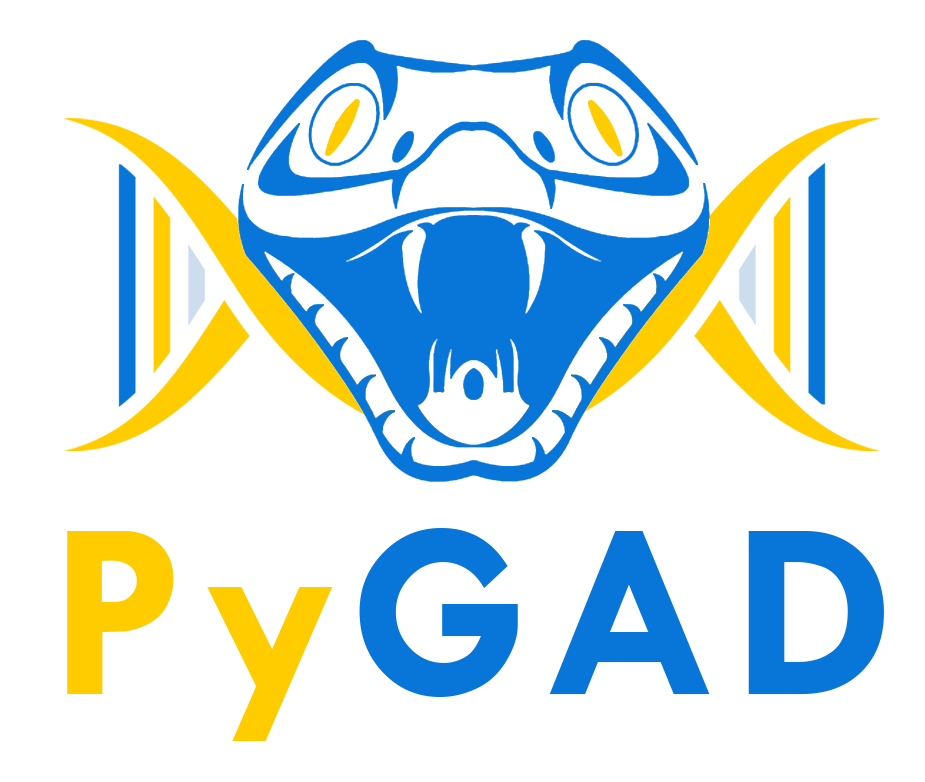PyGAD supports different types of crossover, mutation, and parent selection. PyGAD allows different types of problems to be optimized using the genetic algorithm by customizing the fitness function.

The library is under active development and more features are added regularly. If you want a feature to be supported, please check the Contact Us section to send a request.

# Donation

You can donate via Open Collective: opencollective.com/pygad.

# Installation

Install PyGAD with the following command:

``````pip install pygad
``````

PyGAD is developed in Python 3.7.3 and depends on NumPy for creating and manipulating arrays and Matplotlib for creating figures. The exact NumPy version used in developing PyGAD is 1.16.4. For Matplotlib, the version is 3.1.0.

The source code of the PyGAD' modules is found in the following GitHub projects:

The documentation of the PyGAD library is available at Read The Docs at this link: https://pygad.readthedocs.io. It discusses the modules supported by PyGAD, all its classes, methods, attribute, and functions. For each module, a number of examples are given.

If there is an issue using PyGAD, feel free to post at issue in this GitHub repository https://github.com/ahmedfgad/GeneticAlgorithmPython or by sending an e-mail to ahmed.f.gad@gmail.com.

If you built a project that uses PyGAD, then please drop an e-mail to ahmed.f.gad@gmail.com with the following information so that your project is included in the documentation.

• Project title
• Brief description

The next figure lists the different stages in the lifecycle of an instance of the `pygad.GA` class. Note that PyGAD stops when either all generations are completed or when the function passed to the `on_generation` parameter returns the string `stop`.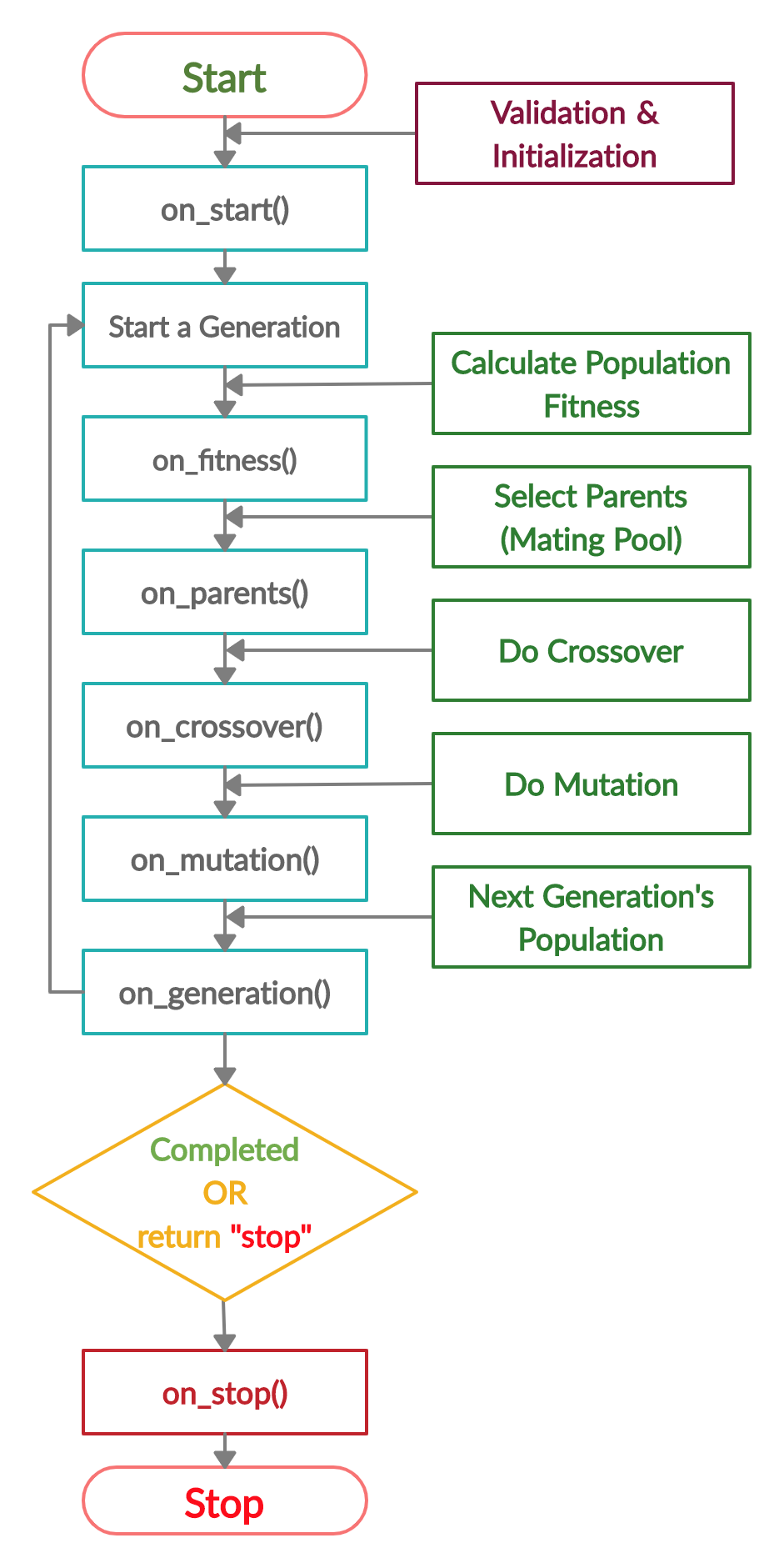The next code implements all the callback functions to trace the execution of the genetic algorithm. Each callback function prints its name.

``````import pygad
import numpy

function_inputs = [4,-2,3.5,5,-11,-4.7]
desired_output = 44

def fitness_func(solution, solution_idx):
output = numpy.sum(solution*function_inputs)
fitness = 1.0 / (numpy.abs(output - desired_output) + 0.000001)
return fitness

fitness_function = fitness_func

def on_start(ga_instance):
print("on_start()")

def on_fitness(ga_instance, population_fitness):
print("on_fitness()")

def on_parents(ga_instance, selected_parents):
print("on_parents()")

def on_crossover(ga_instance, offspring_crossover):
print("on_crossover()")

def on_mutation(ga_instance, offspring_mutation):
print("on_mutation()")

def on_generation(ga_instance):
print("on_generation()")

def on_stop(ga_instance, last_population_fitness):
print("on_stop()")

num_parents_mating=5,
fitness_func=fitness_function,
sol_per_pop=10,
num_genes=len(function_inputs),
on_start=on_start,
on_fitness=on_fitness,
on_parents=on_parents,
on_crossover=on_crossover,
on_mutation=on_mutation,
on_generation=on_generation,
on_stop=on_stop)

ga_instance.run()
``````

Based on the used 3 generations as assigned to the `num_generations` argument, here is the output.

``````on_start()

on_fitness()
on_parents()
on_crossover()
on_mutation()
on_generation()

on_fitness()
on_parents()
on_crossover()
on_mutation()
on_generation()

on_fitness()
on_parents()
on_crossover()
on_mutation()
on_generation()

on_stop()
``````

# Example

Check the PyGAD's documentation for information about the implementation of this example.

``````import pygad
import numpy

"""
Given the following function:
y = f(w1:w6) = w1x1 + w2x2 + w3x3 + w4x4 + w5x5 + 6wx6
where (x1,x2,x3,x4,x5,x6)=(4,-2,3.5,5,-11,-4.7) and y=44
What are the best values for the 6 weights (w1 to w6)? We are going to use the genetic algorithm to optimize this function.
"""

function_inputs = [4,-2,3.5,5,-11,-4.7] # Function inputs.
desired_output = 44 # Function output.

def fitness_func(solution, solution_idx):
# Calculating the fitness value of each solution in the current population.
# The fitness function calulates the sum of products between each input and its corresponding weight.
output = numpy.sum(solution*function_inputs)
fitness = 1.0 / numpy.abs(output - desired_output)
return fitness

fitness_function = fitness_func

num_generations = 100 # Number of generations.
num_parents_mating = 7 # Number of solutions to be selected as parents in the mating pool.

# To prepare the initial population, there are 2 ways:
# 1) Prepare it yourself and pass it to the initial_population parameter. This way is useful when the user wants to start the genetic algorithm with a custom initial population.
# 2) Assign valid integer values to the sol_per_pop and num_genes parameters. If the initial_population parameter exists, then the sol_per_pop and num_genes parameters are useless.
sol_per_pop = 50 # Number of solutions in the population.
num_genes = len(function_inputs)

last_fitness = 0
def callback_generation(ga_instance):
global last_fitness
print("Generation = {generation}".format(generation=ga_instance.generations_completed))
print("Fitness    = {fitness}".format(fitness=ga_instance.best_solution()))
print("Change     = {change}".format(change=ga_instance.best_solution() - last_fitness))
last_fitness = ga_instance.best_solution()

# Creating an instance of the GA class inside the ga module. Some parameters are initialized within the constructor.
num_parents_mating=num_parents_mating,
fitness_func=fitness_function,
sol_per_pop=sol_per_pop,
num_genes=num_genes,
on_generation=callback_generation)

# Running the GA to optimize the parameters of the function.
ga_instance.run()

# After the generations complete, some plots are showed that summarize the how the outputs/fitenss values evolve over generations.
ga_instance.plot_fitness()

# Returning the details of the best solution.
solution, solution_fitness, solution_idx = ga_instance.best_solution()
print("Parameters of the best solution : {solution}".format(solution=solution))
print("Fitness value of the best solution = {solution_fitness}".format(solution_fitness=solution_fitness))
print("Index of the best solution : {solution_idx}".format(solution_idx=solution_idx))

prediction = numpy.sum(numpy.array(function_inputs)*solution)
print("Predicted output based on the best solution : {prediction}".format(prediction=prediction))

if ga_instance.best_solution_generation != -1:
print("Best fitness value reached after {best_solution_generation} generations.".format(best_solution_generation=ga_instance.best_solution_generation))

# Saving the GA instance.
filename = 'genetic' # The filename to which the instance is saved. The name is without extension.
ga_instance.save(filename=filename)

``````

There are different resources that can be used to get started with the genetic algorithm and building it in Python.

## Tutorial: Implementing Genetic Algorithm in Python

To start with coding the genetic algorithm, you can check the tutorial titled Genetic Algorithm Implementation in Python available at these links:

This tutorial is prepared based on a previous version of the project but it still a good resource to start with coding the genetic algorithm.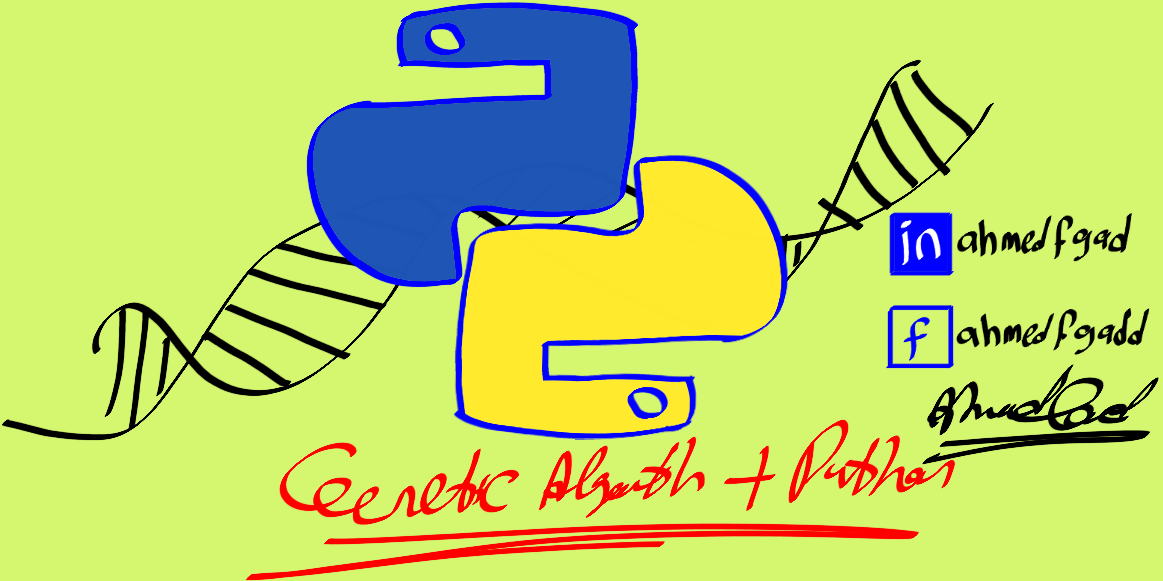## Tutorial: Introduction to Genetic Algorithm

Get started with the genetic algorithm by reading the tutorial titled Introduction to Optimization with Genetic Algorithm which is available at these links: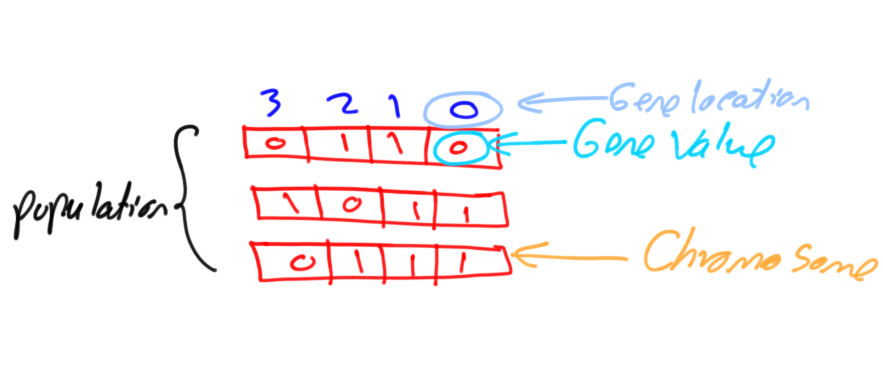## Tutorial: Build Neural Networks in Python

Read about building neural networks in Python through the tutorial titled Artificial Neural Network Implementation using NumPy and Classification of the Fruits360 Image Dataset available at these links: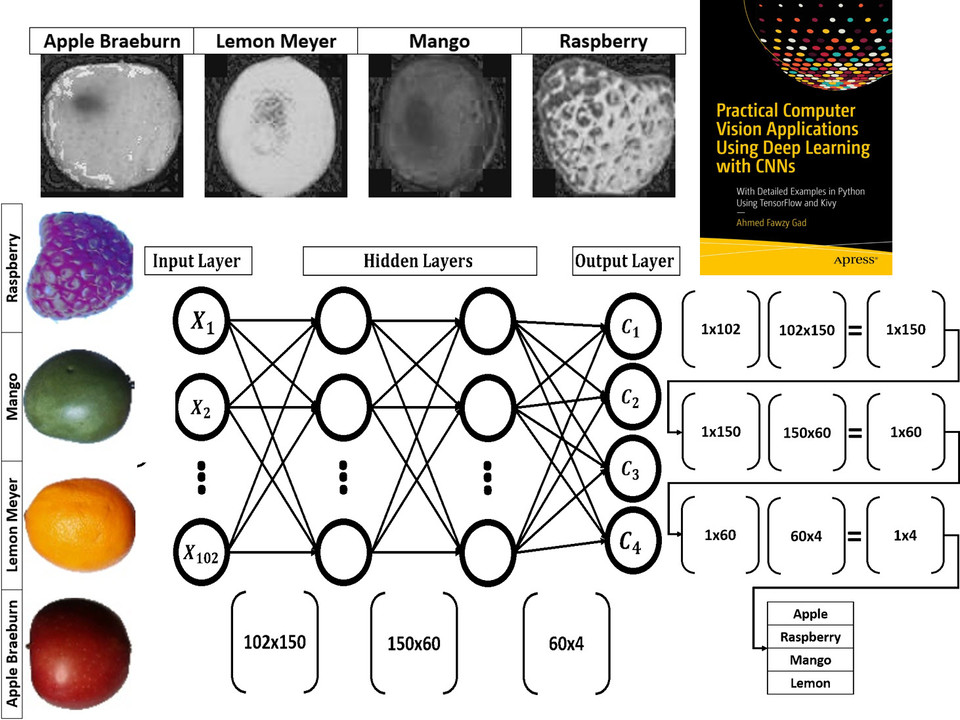## Tutorial: Optimize Neural Networks with Genetic Algorithm

Read about training neural networks using the genetic algorithm through the tutorial titled Artificial Neural Networks Optimization using Genetic Algorithm with Python available at these links: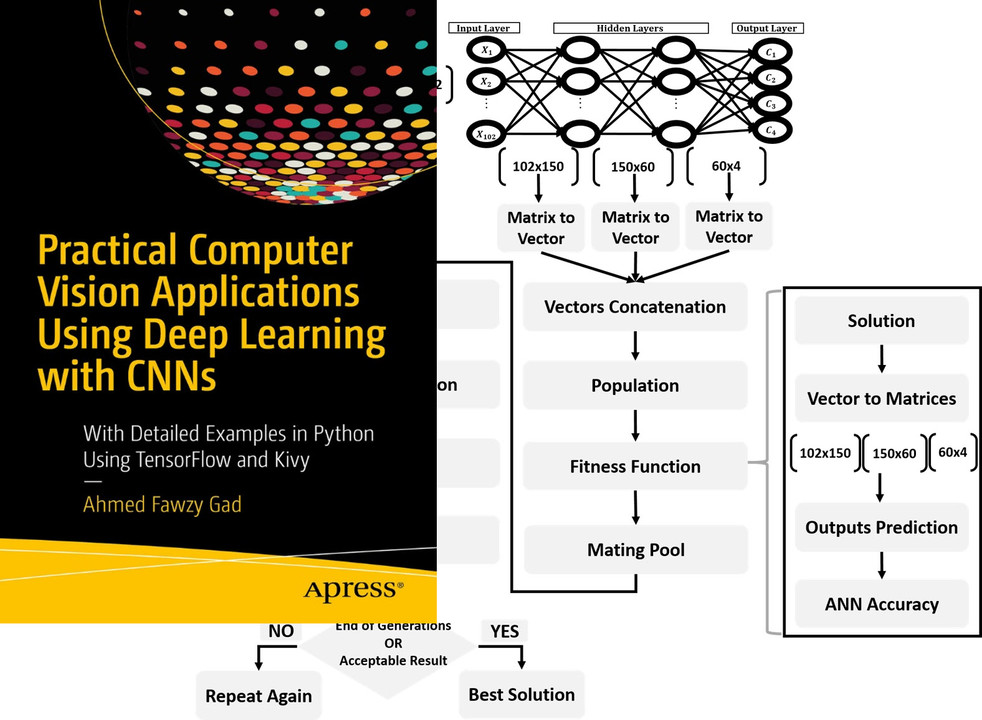## Tutorial: Building CNN in Python

To start with coding the genetic algorithm, you can check the tutorial titled Building Convolutional Neural Network using NumPy from Scratch available at these links:

This tutorial) is prepared based on a previous version of the project but it still a good resource to start with coding CNNs.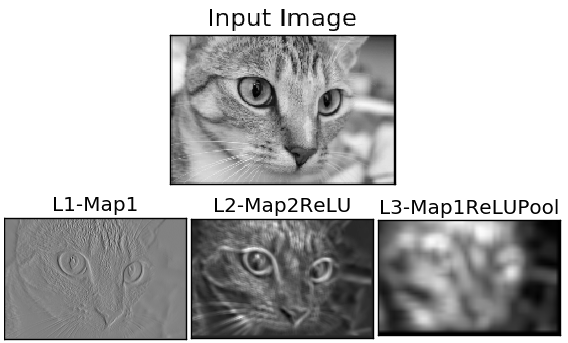## Tutorial: Derivation of CNN from FCNN

Get started with the genetic algorithm by reading the tutorial titled Derivation of Convolutional Neural Network from Fully Connected Network Step-By-Step which is available at these links: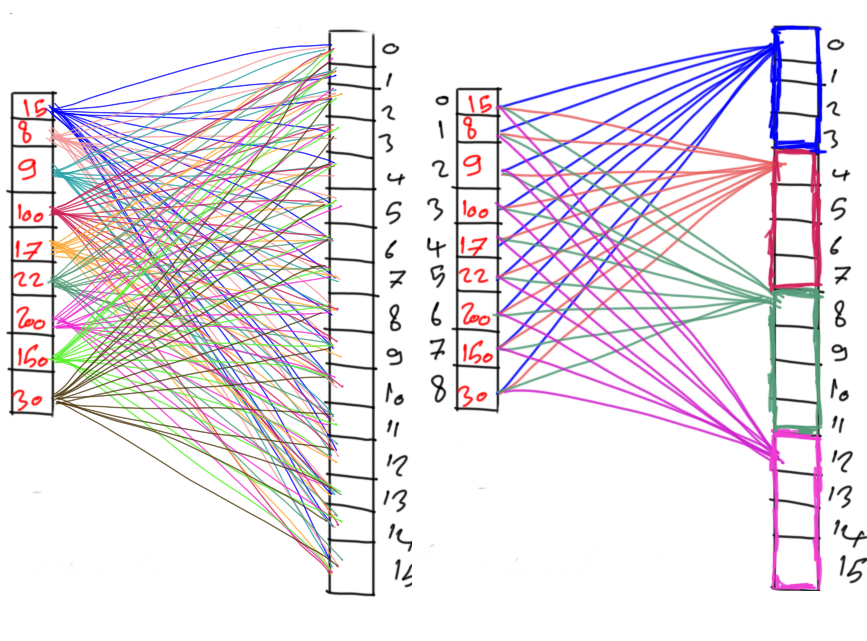## Book: Practical Computer Vision Applications Using Deep Learning with CNNs

You can also check my book cited as Ahmed Fawzy Gad 'Practical Computer Vision Applications Using Deep Learning with CNNs'. Dec. 2018, Apress, 978-1-4842-4167-7 which discusses neural networks, convolutional neural networks, deep learning, genetic algorithm, and more.

Find the book at these links:# Citing PyGAD - Bibtex Formatted Citation

``````@misc{gad2021pygad,
title={PyGAD: An Intuitive Genetic Algorithm Python Library},
year={2021},
eprint={2106.06158},
archivePrefix={arXiv},
primaryClass={cs.NE}
}
``````

## Rate & Review

Great Documentation0
Easy to Use0
Performant0
Highly Customizable0
Bleeding Edge0
Responsive Maintainers0
Poor Documentation0
Hard to Use0
Slow0
Buggy0
Abandoned0
Unwelcoming Community0
100